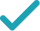Type a math problemSolve for xTo solve the inequality, factor the left hand side. Quadratic polynomial can be factored using the transformation , where and are the solutions of the quadratic equation .
All equations of the form can be solved using the quadratic formula: . Substitute for , for , and for in the quadratic formula.
Do the calculations.
Solve the equation when is plus and when is minus.
Rewrite the inequality by using the obtained solutions.
For the product to be negative, and have to be of the opposite signs. Consider the case when is positive and is negative.
This is false for any .
Consider the case when is positive and is negative.
The solution satisfying both inequalities is .
The final solution is the union of the obtained solutions.
GraphGraph Inequality
Graph Both Sides in 2DGiving is as easy as 1, 2, 3
Get 1,000 points to donate to a school of your choice when you join Give With Bing
x^{2}+3x+2=0
To solve the inequality, factor the left hand side. Quadratic polynomial can be factored using the transformation ax^{2}+bx+c=a\left(x-x_{1}\right)\left(x-x_{2}\right), where x_{1} and x_{2} are the solutions of the quadratic equation ax^{2}+bx+c=0.
x=\frac{-3±\sqrt{3^{2}-4\times 1\times 2}}{2}
All equations of the form ax^{2}+bx+c=0 can be solved using the quadratic formula: \frac{-b±\sqrt{b^{2}-4ac}}{2a}. Substitute 1 for a, 3 for b, and 2 for c in the quadratic formula.
x=\frac{-3±1}{2}
Do the calculations.
x=-1 x=-2
Solve the equation x=\frac{-3±1}{2} when ± is plus and when ± is minus.
\left(x+1\right)\left(x+2\right)<0
Rewrite the inequality by using the obtained solutions.
x+1>0 x+2<0
For the product to be negative, x+1 and x+2 have to be of the opposite signs. Consider the case when x+1 is positive and x+2 is negative.
x\in \emptyset
This is false for any x.
x+2>0 x+1<0
Consider the case when x+2 is positive and x+1 is negative.
x\in \left(-2,-1\right)
The solution satisfying both inequalities is x\in \left(-2,-1\right).
x\in \left(-2,-1\right)
The final solution is the union of the obtained solutions.